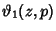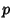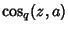## q-Sine

The q-Analog of the Sine function, as advocated by R. W. Gosper, is defined bywhereis a Theta Function andis defined viaThis is a period, Odd Function of unit amplitude with double and triple angle formulas and addition formulas which are analogous to ordinary Sine and Cosine. For example,whereis the q-Cosine, andis q-Pi.

Gosper, R. W. Experiments and Discoveries in-Trigonometry.'' Unpublished manuscript.